## Distance Between Two Cars A car leaves an intersection traveling west. Its position 4 sec later is 19 ft from the intersection. At the same

Question

Distance Between Two Cars A car leaves an intersection traveling west. Its position 4 sec later is 19 ft from the intersection. At the same time, another car leaves the same intersection heading north so that its position 4 sec later is 26 ft from the intersection. If the speeds of the cars at that instant of time are 8 ft/sec and 12 ft/sec, respectively, find the rate at which the distance between the two cars is changing. (Round your answer to one decimal place.)

in progress 0
1 month 2021-08-16T02:31:08+00:00 1 Answers 0 views 0

The answer to the question is;

The rate at which the distance between the two cars is changing is equal to 14.4 ft/sec.

Explanation:

We note that the distance  traveled by each car after 4 seconds is

Car A = 19 ft in the west direction.

Car B = 26 ft in the north direction

The distance between the two cars is given by the length of the hypotenuse side of a right angled triangle with the north being the y coordinate and the  west being the x coordinate.

Therefore, let the distance between the two cars be s

we have

s² = x² + y²

= (19 ft)² + (26 ft)² = 1037 ft²

s =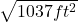= 32.202 ft.

The rate of change of the distance from their location 4 seconds after they commenced their journeys is given by;

Since s² = x² + y² we have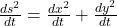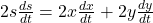which gives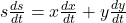We note that the speeds of the cars were given as

Car B moving north = 12 ft/sec, which is the y direction and

Car A moving west = 8 ft/sec which is the x direction.

Therefore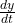=  12 ft/sec and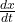= 8 ft/secbecomes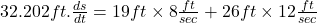= 464 ft²/sec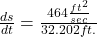= 14.409 ft/sec ≈ 14.4 ft/sec to one place of decimal.